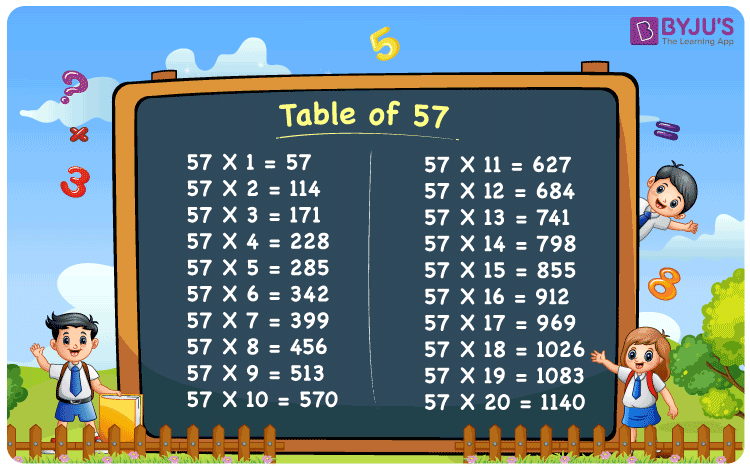# Table of 57

Table of 57 is a multiplication table that represents the repeated addition when 57 is multiplied by natural number. For example, 57 multiplied by 4 is given by 57 + 57 + 57 + 57 = 228. Hence, 57 x 4 = 228. In the same way, we can write the 57 times table up to 20 here in this article. The multiplication table is a very useful tool to solve arithmetic problems, easily. Students can even download the table of 57 from the below-given link.

## Table of 57 Chart## What is 57 times table?

As we already discussed, table of 57 expresses the repeated addition of the number 57, when multiplied by any other number. Let us see, how we can create the 57 times table.

 57 × 1 = 57 57 57 × 2 = 24 57 + 57 = 114 57 × 3 = 36 57 + 57 + 57 = 171 57 × 4 = 48 57 + 57 + 57 + 57 = 228 57 × 5 = 60 57 + 57 + 57 + 57 + 57 = 285 57 × 6 = 72 57+ 57+ 57+ 57+ 57+ 57= 342 57 × 7 = 84 57+ 57+ 57+ 57+ 57+ 57+ 57= 399 57 × 8 = 96 57+ 57+ 57+ 57+ 57+ 57+ 57+ 57= 456 57 × 9 = 108 57+ 57+ 57+ 57+ 57+ 57+ 57+ 57+ 57= 513 57 × 10 = 570 57+ 57+ 57+ 57+ 57+ 57+ 57+ 57+ 57+ 57 = 570

## Multiplication Table of 57

Find the below table of 57 up to 20 times. This table can be used for solving multiplication problems.

 57 × 1 = 57 57 × 2 = 114 57 × 3 = 171 57 × 4 = 228 57 × 5 = 285 57 × 6 = 342 57 × 7 = 399 57 × 8 = 456 57 × 9 = 513 57 × 10 = 570 57 × 11 = 627 57 × 12 = 684 57 × 13 = 741 57 × 14 = 798 57 × 15 = 855 57 × 16 = 912 57 × 17 = 969 57 × 18 = 1026 57 × 19 = 1083 57 × 20 = 1140

## Solved Examples on Table of 57

Q.1: Find the value of 57 x 4 + 60.

Solution: Given,

57 x 4 + 60

= 228 + 60

= 288

Q.2: Evaluate: 1000 – 57 x 10.

Solution: 1000 – 57 x 10

= 1000 – 570

= 430

## Frequently Asked Questions on Table of 57

### What is table of 57?

Table of 57 is the multiplication table that represents the 57 times of a number. It is helpful in performing mathematical calculations.

### What are 57 times 3?

The value of 57 times 3 is 57 × 3 = 171.

### 57 times of which number is equal to 741?

From the table of 57, 57 times 13 is equal to 741.
57 x 13 = 741

### What is 57 multiplied by 11?

57 multiplied by 11 is 627. From the table of 57,
57 x 11 = 627